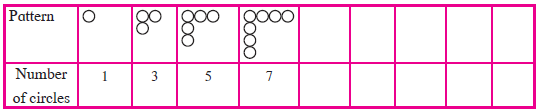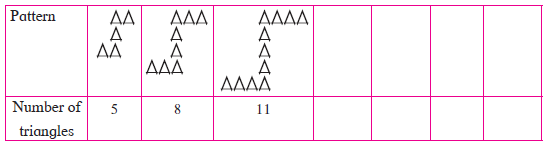Tamil Nadu Board of Secondary EducationSSLC (English Medium) Class 10th

# Sequence

## Definition

A set of numbers where the numbers are arranged in a definite order, like the natural numbers, is called a sequence.

## Notes

We write numbers 1, 2, 3, 4, . . . in an order. In this order we can tell the position of any number. For example,  number 13 is at 13th position. The numbers 1, 4, 9, 16, 25, 36, 49, . . . are also written in a particular order. Here 16 = 42 is at 4th position. similarly, 25 = 52 is at the 5th position; 49 = 72 is at the 7th position. In this set of numbers also, place of each number is detremined.

In a sequence a particular number is written at a particular position. If the numbers are written as a1,a2,a3,a4,..... then a1 is first, a2 is second, . . . and so on. It is clear that an is at the nth place. A sequence of the numbers is also represented by alphabets f1, f2, f3, . . . and we find that there is a definite order in which numbers are arranged.When students stand in a row for drill on the playground they form a sequence.We have experienced that some sequences have a particular pattern.Complete the given patternLook at the patterns of the numbers. Try to find a rule to obtain the next number from its preceding number. This helps us to write all the next numbers. See the numbers 2, 11, -6, 0, 5, -37, 8, 2, 61 written in this order.
Here a1 = 2, a2 = 11, a3 = -6, . . . This list of numbers is also a sequence. But in this case we cannot tell why a particular term is at a particular position ; similarly we cannot tell a definite relation between the consecutive terms.
In general, only those sequences are studied where there is a rule which determines the next term.

For example (1) 4, 8, 12, 16 . . . (2) 2, 4, 8, 16, 32, . . . (3)1/5,1/10,1/15,1/20,........

If you would like to contribute notes or other learning material, please submit them using the button below.